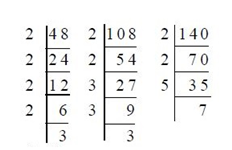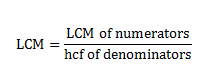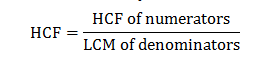New Students Offer - Use Code HELLO

# LCM and HCF Short Tricks with Examples## LCM (LEAST COMMON MULTIPLE)

The LCM is the least number which is exactly divisible by each one of the given numbers.

## Methods of LCM

### Factorization method:

Express each one of the given numbers as the product of prime factors. The product of the highest powers of all the factors gives LCM.
Ex: Find the LCM of (48,108,140)⇒108=2²*3³
⇒48=2⁴*3
⇒140=2²*5*7

### Division method

Ex: Find the LCM of (16,24,36,54)
⇒LCM=2⁴ ⅹ 3³ = 432 is the answer

Learn Fast Maths Trick here

## Types of LCM

LCM of integers: We have to do the same normal calculation as above
LCM of decimals: We have to convert decimal into integers by making the number of digits after decimal point is common, now find LCM normally and add decimal point

Example
⇒LCM of (0.16,0.48)
⇒Now the decimal is converted and we get is (16,48)
⇒Now do LCM and we get the answer =2⁴*3=48
⇒Now the number 48 is converted into decimal 0.48

LCM of fractions
The LCM of fractions can be done by formula## The remainder based problem in LCM

When the remainder is same, i.e. “R”

Shortcut ⇢ LCM [n1,n2,n3]+ R

### Example

Find the LCM of (8,9,12,15) that leaves same remainder 1 in each the case.
Solution:
⇒LCM of [8,9,12,15]
⇒2³*3²*5
⇒360
⇒à+1
⇒Now we get the answer as 361

When the remainder is different in each case

Shortcut 1⇢ n1- R1 = n2-R2 = n3-R3 = R
Shortcut 2⇢ LCM(n1, n2, n3) - R

### Example

Find the LCM of (18, 27, 36) that leaves different remainders (5, 14, 23)
Solution:
⇒18-5=13
⇒27-14=13
⇒36-23=13
⇒So now R=13
⇒LCM (18, 27, 36)=3³*2²
⇒108
⇒Now we subtract the number 108 with R⇢ [108-13=95]

## Exercise with Solution

Ques 1. Find the smallest number which when diminished by 7 is divisible by 12,16,18,21 and 28?
Solution
We have to use the same remainder formula and the answer is 1015

Ques 2: Find the least number which when divided by 48,60,72,108and 140 leaves 38,50,62,98 and 130 as remainders respectively .
Solution
We have to use different remainder formula and the answer is 15110

3) The largest four digit number which when divided by 4,7 or 13 leaves a remainder of 3 in each case?
Solution
⇒ The largest 4 digit no is 9999
⇒ LCM of 4,7,13 is 364
⇒ Now divide 9999 by 364 and we get the remainder as 171
⇒ 4 digit divisible by 4,7,13=(9999-171)=9828
⇒ Now add the remainder 3 given in question (9828+3)

Ques4. Six bells commence tolling together and toll at intervals of 2,4,6,8,10 and 12 seconds respectively. In 30 minutes how many times do they toll together?
Solution
⇒ Take LCM of 2,4,6,8,10 and 12 is 120
⇒ So the bell will toll together every 120sec = 2min
⇒ In 30 minutes they will toll together (30/2)+1
⇒ And the answer is 16 times.

Ques 5. Traffic lights at three different road crossing change after every 48 sec, 72sec, and 108sec respectively. If they all change simultaneously at 8:20:00 hours then they will again change simultaneously at what time?
Solution
⇒ Take LCM of 48,72,108 is 432 sec
⇒ Now 432 sec =7 min 12 sec
⇒ Now add the time (8hr 20min 0sec)+(7min 12 sec)
⇒ so signal will again change simultaneously at 8:27:12.

## Highest Common Factor (HCF)

The HCF of two or more than two numbers is the greatest number that divides each of them exactly. The HCF can found in the following two ways

### Prime factorization method:

Express each one of the given numbers as the product of prime factors. The product of least powers of common prime factor gives HCF
Example
Find the HCF of (36,48)
⇒ 36=2² х 3²
⇒ 48=2⁴ х 3
⇒ So 2² х 3 is the common least factor and the answer is 12

### Long division method

Example
Find the HCF of (30,18)## Types of HCF

HCF of integers: We have to do the same normal calculation as above

HCF of decimals: We have to convert decimal into integers by making the number of digits after decimal point is common

Example
(0.54,0.06,1.08)
⇨Let us multiply each number by 100
⇨We get 54, 6 and 108
⇨Now
⇨The HCF of 54, 6 and 108 = 6
⇨Now divide 6 by 100
⇨We get 0.06
⇨ So the answer is 0.06

HCF of fractions: The HCF of fractions can be done by the following formula## Remainder based problems in HCF

### Case 1: Same remainder in each case

Shortcut➡HCF [(n2-n1)(n3-n2)(n3-n1)]

Example
Find the greatest number that can divide (62,132,237) that leaves same remainder in each case
Solution:
➡HCF of [(132-62)(237-132)(237-62)]
➡HCF of [70,105,175]
➡Now we take HCF of these numbers and we get the answer as 35.

### Case 2: Same remainder “R”

Shortcut⇨HCF [(n1-R)(n2-R)(n3-R)]
Example
Find the HCF of (201,333,339) that leaves same remainder 3 in each the case
Solution:
➡HCF of [(201-3)(333-3)(339-3)]
➡HCF of[(198)(330)(396)]
➡Now we take HCF of these numbers and we get the answer as 66

### Case 2: Different remainder in each case

Shortcut⇨ HCF [(n1-R1)(n2-R2)(n3-R3)]

Example
Find the HCF of (42,49,56) that leaves different remainders(0,1,2)
Solution:
➡ HCF of [(42-0) (49-1) (56-2)]
➡ HCF of [42, 48, 54]
➡ Now we take HCF of these numbers and we get the answer as 6.

## Ratio Based Problems

### Formula

Example
A and B are in the ratio 2:3. If their HCF is 13, then find the values of A and B?
Solution:
➡Now we apply the formula 2:3 = Value/13
➡Value =13*2, 13*3
➡Value of A and B =26, 39

## Problems with Solutions

Ques 1:  Two numbers are in the ratio 4:7 and their HCF is 15. Find the difference between the two numbers?
Solution:  We have to use ratio formula and we will get two numbers 60,105 and its difference is 45

Ques 2: Find the largest number which divides 52,100, 72 to leave the same remainder?
Solution:  Use the same remainder formula and we get answer as 4

Ques 3: Find the greatest number which on dividing 1657 and 2037, leaves a remainder of 6 and 5 respectively.
Solution: Use same remainder for each formula and we get 127

Ques 4: The sum of two numbers is 216 and their HCF is 27. How many pairs of such numbers are there?
Solution:
➡In this two numbers x and y and hcf is 27
➡27x+27y=216
➡27(x+y)=216
➡x+y=216/27
➡x+y=8
➡Now we check prime multiplication and it is
➡7+1=8 ⇾1st pair coprime
➡5+3=8⇾2nd pair
➡4+4=8⇾ not right pair

Ques 5:  Which greatest possible length can be used to measure exactly 15meter 75cm, 11meter 25cm and 7meter 65 cm?
Solution: It is just decimal problem and answer is 45

Ques 6: Three different containers contain 496 liters , 403 liters and 713 liters of mixture of milk and water respectively. What biggest measure all the different quantities exactly?
Solution: See which number divides three terms and gives 0 and answer is 31

Ques 7: Find the maximum number of students among them 1001 pens and 910 pencils can be distributed in such a way that each student gets the same number of pens and same number of pencils?
Solution: HCF of 1001 and 910 and answer is 91.